# Forward–backward quasi-Newton methods for nonsmooth optimization problems

## Abstract

The forward–backward splitting method (FBS) for minimizing a nonsmooth composite function can be interpreted as a (variable-metric) gradient method over a continuously differentiable function which we call forward–backward envelope (FBE). This allows to extend algorithms for smooth unconstrained optimization and apply them to nonsmooth (possibly constrained) problems. Since the FBE can be computed by simply evaluating forward–backward steps, the resulting methods rely on a similar black-box oracle as FBS. We propose an algorithmic scheme that enjoys the same global convergence properties of FBS when the problem is convex, or when the objective function possesses the Kurdyka–Łojasiewicz property at its critical points. Moreover, when using quasi-Newton directions the proposed method achieves superlinear convergence provided that usual second-order sufficiency conditions on the FBE hold at the limit point of the generated sequence. Such conditions translate into milder requirements on the original function involving generalized second-order differentiability. We show that BFGS fits our framework and that the limited-memory variant L-BFGS is well suited for large-scale problems, greatly outperforming FBS or its accelerated version in practice, as well as ADMM and other problem-specific solvers. The analysis of superlinear convergence is based on an extension of the Dennis and Moré theorem for the proposed algorithmic scheme.

This is a preview of subscription content, access via your institution.

## References

1. Moreau, J.-J.: Proximité et dualité dans un espace Hilbertien. Bulletin de la Société mathématique de France 93, 273–299 (1965)

2. Lions, P.-L., Mercier, B.: Splitting algorithms for the sum of two nonlinear operators. SIAM J. Numer. Anal. 16(6), 964–979 (1979)

3. Combettes, P.L., Pesquet, J.-C.: Proximal splitting methods in signal processing. In: Fixed-Point Algorithms for Inverse Problems in Science and Engineering, pp. 185–212. Springer (2011)

4. Łojasiewicz, S.: Une propriété topologique des sous-ensembles analytiques réels. Les équations aux dérivées partielles, pp. 87–89 (1963)

5. Łojasiewicz, S.: Sur la géométrie semi- et sous-analytique. Annales de l’institut Fourier 43(5), 1575–1595 (1993)

6. Kurdyka, K.: On gradients of functions definable in o-minimal structures. Annales de l’institut Fourier 48(3), 769–783 (1998)

7. Attouch, H., Bolte, J., Svaiter, B.F.: Convergence of descent methods for semi-algebraic and tame problems: proximal algorithms, forward-backward splitting, and regularized Gauss–Seidel methods. Math. Program. 137(1–2), 91–129 (2013)

8. Attouch, H., Bolte, J.: On the convergence of the proximal algorithm for nonsmooth functions involving analytic features. Math. Program. 116(1), 5–16 (2009)

9. Attouch, H., Bolte, J., Redont, P., Soubeyran, A.: Proximal alternating minimization and projection methods for nonconvex problems: an approach based on the Kurdyka–Łojasiewicz inequality. Math. Oper. Res. 35(2), 438–457 (2010)

10. Bolte, J., Sabach, S., Teboulle, M.: Proximal alternating linearized minimization for nonconvex and nonsmooth problems. Math. Program. 146(1), 459–494 (2014)

11. Ochs, P., Chen, Y., Brox, T., Pock, T.: iPiano: inertial proximal algorithm for nonconvex optimization. SIAM J. Imaging Sci. 7(2), 1388–1419 (2014)

12. Nesterov, Y.: A method for solving the convex programming problem with convergence rate $$O(1/k^2)$$. Doklady Akademii Nauk SSSR 269(3), 543–547 (1983)

13. Tseng, P.: On accelerated proximal gradient methods for convex-concave optimization. Department of Mathematics, University of Washington, Tech. Rep. (2008)

14. Beck, A., Teboulle, M.: A fast iterative shrinkage-thresholding algorithm for linear inverse problems. SIAM J. Imaging Sci. 2(1), 183–202 (2009)

15. Nesterov, Y.: Gradient methods for minimizing composite functions. Math. Program. 140(1), 125–161 (2013)

16. Becker, S., Fadili, J.: A quasi-Newton proximal splitting method. In: Advances in Neural Information Processing Systems, pp. 2618–2626 (2012)

17. Lee, J., Sun, Y., Saunders, M.: Proximal Newton-type methods for convex optimization. In: Advances in Neural Information Processing Systems, pp. 836–844 (2012)

18. Scheinberg, K., Tang, X.: Practical inexact proximal quasi-Newton method with global complexity analysis. Math. Program. 160(1), 495–529 (2016)

19. Patrinos, P., Bemporad, A.: Proximal Newton methods for convex composite optimization. In: IEEE Conference on Decision and Control, pp. 2358–2363 (2013)

20. Fukushima, M.: Equivalent differentiable optimization problems and descent methods for asymmetric variational inequality problems. Math. Program. 53(1), 99–110 (1992)

21. Yamashita, N., Taji, K., Fukushima, M.: Unconstrained optimization reformulations of variational inequality problems. J. Optim. Theory Appl. 92(3), 439–456 (1997)

22. Facchinei, F., Pang, J.-S.: Finite-Dimensional Variational Inequalities and Complementarity Problems, vol. II. Springer, Berlin (2003)

23. Li, W., Peng, J.: Exact penalty functions for constrained minimization problems via regularized gap function for variational inequalities. J. Glob. Optim. 37, 85–94 (2007)

24. Patrinos, P., Sopasakis, P., Sarimveis, H.: A global piecewise smooth Newton method for fast large-scale model predictive control. Automatica 47, 2016–2022 (2011)

25. Liu, T., Pong, T.K.: Further properties of the forward–backward envelope with applications to difference-of-convex programming. Computational Optimization and Applications, pp. 1–32, 2017. doi:10.1007/s10589-017-9900-2

26. Dennis, J.E., Moré, J.J.: A characterization of superlinear convergence and its application to quasi-Newton methods. Math. Comput. 28(126), 549–560 (1974)

27. Dai, Y.-H.: Convergence properties of the BFGS algorithm. SIAM J. Optim. 13(3), 693–701 (2002)

28. Mascarenhas, W.F.: The BFGS method with exact line searches fails for non-convex objective functions. Math. Program. 99(1), 49–61 (2004)

29. Mascarenhas, W.F.: On the divergence of line search methods. Comput. Appl. Math. 26, 129–169 (2007)

30. Dai, Y.H.: A perfect example for the BFGS method. Math. Program. 138, 501–530 (2013)

31. Rockafellar, R.T., Wets, R.J.-B.: Variational Analysis, vol. 317. Springer, Berlin (2011)

32. Bauschke, H.H., Combettes, P.L.: Convex Analysis and Monotone Operator Theory in Hilbert Spaces. Springer, Berlin (2011)

33. Combettes, P.L., Wajs, V.R.: Signal recovery by proximal forward–backward splitting. Multiscale Model. Simul. 4(4), 1168–1200 (2005)

34. Dennis, J.E., Schnabel, R.B.: Numerical Methods for Unconstrained Optimization and Nonlinear Equations. Society for Industrial and Applied Mathematics (1996).

35. Bertsekas, D.: Nonlinear Programming. Athena Scientific, Belmont (1999)

36. Lemaréchal, C., Sagastizábal, C.: Practical aspects of the Moreau–Yosida regularization: theoretical preliminaries. SIAM J. Optim. 7(2), 367–385 (1997)

37. Bernstein, D.S.: Matrix Mathematics: Theory, Facts, and Formulas with Application to Linear Systems Theory. Princeton University Press, Woodstock (2009)

38. Rockafellar, R.T.: First- and second-order epi-differentiability in nonlinear programming. Trans. Am. Math. Soc. 307, 75–108 (1988)

39. Rockafellar, R.: Second-order optimality conditions in nonlinear programming obtained by way of epi-derivatives. Math. Oper. Res. 14(3), 462–484 (1989)

40. Poliquin, R.A., Rockafellar, R.T.: Amenable functions in optimization. In: Giannessi, F. (ed.) Nonsmooth Optimization: Methods and Applications, pp. 338–353. Gordon and Breach (1992).

41. Poliquin, R.A., Rockafellar, R.T.: Second-order nonsmooth analysis in nonlinear programming. In: Du, D., Qi, L., Womersley, R. (eds.) Recent Advances in Nonsmooth Optimization, pp. 322–350. World Scientific Publishers (1995)

42. Rockafellar, R.T.: Monotone operators and the proximal point algorithm. SIAM J. Control Optim. 14(5), 877–898 (1976)

43. Fukushima, M., Qi, L.: A globally and superlinearly convergent algorithm for nonsmooth convex minimization. SIAM J. Optim. 6(4), 1106–1120 (1996)

44. Bonnans, J.F., Gilbert, J.C., Lemaréchal, C., Sagastizábal, C.A.: A family of variable metric proximal methods. Math. Program. 68(1), 15–47 (1995)

45. Mifflin, R., Sun, D., Qi, L.: Quasi-Newton bundle-type methods for nondifferentiable convex optimization. SIAM J. Optim. 8(2), 583–603 (1998)

46. Chen, X., Fukushima, M.: Proximal quasi-Newton methods for nondifferentiable convex optimization. Math. Program. 85(2), 313–334 (1999)

47. Burke, J.V., Qian, M.: On the superlinear convergence of the variable metric proximal point algorithm using Broyden and BFGS matrix secant updating. Math. Program. 88(1), 157–181 (2000)

48. Sagara, N., Fukushima, M.: A trust region method for nonsmooth convex optimization. J. Ind. Manage. Optim. 1(2), 171–180 (2005)

49. Squire, W., Trapp, G.: Using complex variables to estimate derivatives of real functions. SIAM Rev. 40(1), 110–112 (1998)

50. Nocedal, J., Wright, S.: Numerical Optimization. Springer, Berlin (2006)

51. Noll, D., Rondepierre, A.: Convergence of linesearch and trust-region methods using the Kurdyka–Łojasiewicz inequality. In: Bailey, D.H., Bauschke, H.H., Borwein, P., Garvan, F., Théra, M., Vanderwerff, J.D., Wolkowicz, H. (eds.) Computational and Analytical Mathematics: In Honor of Jonathan Borwein’s 60th birthday, pp. 593–611. Springer, New York (2013)

52. Bolte, J., Daniilidis, A., Lewis, A.: The Łojasiewicz inequality for nonsmooth subanalytic functions with applications to subgradient dynamical systems. SIAM J. Optim. 17(4), 1205–1223 (2007)

53. Dennis, J.E., Moré, J.J.: Quasi-Newton methods, motivation and theory. SIAM Rev. 19(1), 46–89 (1977)

54. Powell, M.J.D.: Some global convergence properties of a variable metric algorithm for minimization without exact line searches. In: Cottle, R.W., Lemke, C.E. (eds.) Nonlinear Programming. SIAM-AMS Proceedings 9, pp. 53–72. American Mathematical Society (1976)

55. Liu, D.C., Nocedal, J.: On the limited memory BFGS method for large scale optimization. Math. Program. 45(1), 503–528 (1989)

56. Ip, C.-M., Kyparisis, J.: Local convergence of quasi-Newton methods for B-differentiable equations. Math. Program. 56(1–3), 71–89 (1992)

57. Nocedal, J.: Updating quasi-Newton matrices with limited storage. Math. Comput. 35(151), 773–782 (1980)

58. Li, D.-H., Fukushima, M.: On the global convergence of the BFGS method for nonconvex unconstrained optimization problems. SIAM J. Optim. 11(4), 1054–1064 (2001)

59. Hiriart-Urruty, J.-B., Lemaréchal, C.: Convex Analysis and Minimization Algorithms I: Fundamentals, vol. 305. Springer, Berlin (1996)

60. Fletcher, R.: Practical Methods of Optimization. Wiley, Hoboken (1987)

61. Dai, Y.-H., Yuan, Y.: A nonlinear conjugate gradient method with a strong global convergence property. SIAM J. Optim. 10(1), 177–182 (1999)

62. Wright, S.J., Nowak, R.D., Figueiredo, M.A.: Sparse reconstruction by separable approximation. IEEE Trans. Signal Process. 57(7), 2479–2493 (2009)

63. Wen, Z., Yin, W., Goldfarb, D., Zhang, Y.: A fast algorithm for sparse reconstruction based on shrinkage, subspace optimization, and continuation. SIAM J. Sci. Comput. 32(4), 1832–1857 (2010)

64. Candès, E.J., Recht, B.: Exact matrix completion via convex optimization. Found. Comput. Math. 9(6), 717–772 (2009)

65. Toh, K.-C., Yun, S.: An accelerated proximal gradient algorithm for nuclear norm regularized linear least squares problems. Pac. J. Optim. 6(3), 615–640 (2010)

66. Boţ, R.I., Csetnek, E.R., László, S.C.: An inertial forward-backward algorithm for the minimization of the sum of two nonconvex functions. EURO J. Comput. Optim. 4(1), 3–25 (2016)

67. Pang, J.-S.: Newton’s method for B-differentiable equations. Math. Oper. Res. 15(2), 311–341 (1990)

68. Poliquin, R., Rockafellar, R.: Generalized Hessian properties of regularized nonsmooth functions. SIAM J. Optim. 6(4), 1121–1137 (1996)

69. Horn, R.A., Johnson, C.R.: Matrix Analysis. Cambridge University Press, Cambridge (2012)

70. Byrd, R.H., Nocedal, J.: A tool for the analysis of quasi-Newton methods with application to unconstrained minimization. SIAM J. Numer. Anal. 26(3), 727–739 (1989)

## Author information

Authors

### Corresponding author

Correspondence to Lorenzo Stella.

This work was supported by the KU Leuven Research Council under BOF/STG-15-043.

## Appendices

### Appendix 1: Definitions and known results

We say that $$G:\mathbb {R}^n\rightarrow \mathbb {R}^m$$ is strictly continuous at $$\bar{x}$$ if [31, Def. 9.1(b)]

\begin{aligned} \limsup _{ \begin{array}{c} (x,y)\rightarrow (\bar{x},\bar{x})\\ x\ne y \end{array} }{ \frac{\Vert G(y)-G(x)\Vert }{\Vert y-x\Vert } } {}<{} \infty . \end{aligned}

If G is differentiable, we letdenote the Jacobian of G. When $$m=1$$ we indicate withthe gradient of G and withits Hessian, whenever it makes sense. We say that G is strictly differentiable at $$\bar{x}$$ if it satisfies the stronger limit [31, Eq. 9(7)]The next result states that strict differentiability is preserved by composition; its proof is a trivial computation and is therefore omitted.

### Proposition 6.1

Let $$F:\mathbb {R}^n\rightarrow \mathbb {R}^m$$, $$P:\mathbb {R}^m\rightarrow \mathbb {R}^k$$. Suppose that F and P are (strictly) differentiable at $$\bar{x}$$ and $$F(\bar{x})$$, respectively. Then the composition $$T=P\circ F$$ is (strictly) differentiable at $$\bar{x}$$.

Similarly, the product of (strictly) differentiable functions is still (strictly) differentiable. However, if one of the two functions vanishes at one point, then we may relax some assumptions, as it is proved in the next result.

### Proposition 6.2

Let $$Q:\mathbb {R}^n\rightarrow \mathbb {R}^{m\times k}$$ and $$R:\mathbb {R}^n\rightarrow \mathbb {R}^k$$, and suppose that $$R(\bar{x}) = 0$$. If Q is (strictly) continuous at $$\bar{x}$$ and R is (strictly) differentiable at $$\bar{x}$$, then their product $$G:\mathbb {R}^n \rightarrow \mathbb {R}^m$$ defined as $$G(x) = Q(x)R(x)$$ is (strictly) differentiable at $$\bar{x}$$ with.

### Proof

Suppose first that Q is continuous at $$\bar{x}$$ and R is differentiable at $$\bar{x}$$. Then, expanding R(x) at $$\bar{x}$$ and since $$G(\bar{x})=0$$, we obtain

The quantityis bounded, and continuity of Q at $$\bar{x}$$ implies that taking the limit for $$\bar{x}\ne x\rightarrow \bar{x}$$ yields 0. This proves that G is differentiable at $$\bar{x}$$.

Suppose now that Q is strictly continuous at $$\bar{x}$$, and that R is strictly differentiable at $$\bar{x}$$. Then, expanding R(y) at x we obtain

The quantityis bounded, and by strict continuity of Q at $$\bar{x}$$ so is $$\frac{Q(x)-Q(y)}{\Vert x-y\Vert }$$ for xy sufficiently close to $$\bar{x}$$. Taking the limit for $$(x,y)\rightarrow (\bar{x},\bar{x})$$ with $$x\ne y$$ in the above expression then yields 0, proving strict differentiability. Uniqueness of the Jacobian proves also the claimed form of. $$\square$$

### Definition 6.3

A mapping $$G:\mathbb {R}^n\rightarrow \mathbb {R}^m$$ is said to be semidifferentiable (or B -differentiable [56, 67]) at a point $$\bar{x}\in \mathbb {R}^n$$ if there exists a positively homogeneous mappingsuch thatIt is strictly semidifferentiable at $$\bar{x}$$ if the stronger limit holdsis called semiderivative of G at $$\bar{x}$$. If G is (strictly) semidifferentiable at every point of a set S, then it is said to be (strictly) semidifferentiable in S.

### Proposition 6.4

([67, Thm. 2]) Suppose that $$G:\mathbb {R}^n\rightarrow \mathbb {R}^m$$ is semidifferentiable in a neighborhood of $$\bar{x}\in \mathbb {R}^n$$. Then, the following are equivalent:

1. (a)is continuous in its first argument at $$\bar{x}$$ for all $$d\in \mathbb {R}^n$$;

2. (b)

G is strictly semidifferentiable at $$\bar{x}$$;

3. (c)

G is strictly (Fréchet) differentiable at $$\bar{x}$$.

### Proposition 6.5

Suppose that $$G:\mathbb {R}^n\rightarrow \mathbb {R}^m$$ is semidifferentiable in a neighborhood N of $$\bar{x}$$ and thatis calm at $$\bar{x}$$, i.e., there exists $$L>0$$ such that, for all $$x\in N$$ and $$d\in \mathbb {R}^n$$ with $$\Vert d\Vert =1$$,Then,### Proof

Follows from [56, Lem. 2.2] by observing that the assumption of Lipschitz-continuity may be relaxed to calmness. $$\square$$

### Proof of Lemma 2.9

We know from [68, Thm.s 3.8, 4.1] that $$\mathop {\mathrm{prox}}\nolimits _{\gamma g}$$ is (strictly) differentiable at $$x-\gamma \nabla f(x)$$ if and only if g satisfies Assumption 4 (Assumption 5) at x for $$-\nabla f(x)$$. Since $$f\in C^2$$ by assumption, then in particular $$\nabla f$$ is strictly differentiable. The formula (2.7) follows from Proposition 6.1 with $$P = \mathop {\mathrm{prox}}\nolimits _{\gamma g}$$ and $$F(x) = x - \gamma \nabla f(x)$$.

Matrix $$Q_{\gamma }(x)$$ is symmetric since $$f\in C^2$$ and positive definite if $$\gamma < 1/L_f$$. To obtain an expression forwe can apply [31, Ex. 13.45] to the tilted function $$g+\langle \nabla f(x),{}\cdot {} \rangle$$ so that, lettingandthe idempotent and symmetric projection matrix on S,

where $$^\dagger$$ indicates the pseudo-inverse, and last equality is due to [37, Facts 6.4.12(i)-(ii) and 6.1.6(xxxii)] and the properties of M as stated in Assumption 4. Apparently $$P_{\gamma }(x)\succeq 0$$ is symmetric and $$\Vert P_{\gamma }(x)\Vert \le 1$$. $$\square$$

### Proof of Theorem 2.11

If follows from Theorem 2.10 that the Hessian $$\nabla ^2\varphi _{\gamma }(x)$$ exists and is symmetric. Moreover, from [31, Ex. 13.18] we know that for all $$d\in \mathbb {R}^n$$(6.1)

2.11(a) $$\Leftrightarrow$$ 2.11(b): Follows directly from (6.1), using [31, Thm. 13.24(c)].

2.11(c) $$\Leftrightarrow$$ 2.11(d): Letting $$Q = Q_{\gamma }(x)$$, we see from (2.7) and (2.9) thatis similar to the symmetric matrix $$Q^{-1/2}\nabla ^2\varphi _{\gamma }(x)Q^{-1/2}$$, which is positive definite if and only if $$\nabla ^2\varphi _{\gamma }(x)$$ is.

2.11(b) $$\Leftrightarrow$$ 2.11(c): From the point above we know thathas all real eigenvalues, and it can be easily seen to be similar to $$\gamma ^{-1}(I-QP)$$, where $$P = P_{\gamma }(x)$$. From [69, Thm. 7.7.3] it follows that $$\lambda _{\min }(I-QP) > 0$$ if and only if $$Q^{-1} \succ P$$. For all $$d\in S$$, using (2.8) we have

\begin{aligned} \langle d,(Q^{-1}-P)d \rangle {}={}&\langle d,Q^{-1}d \rangle {}-{} \langle d, \Pi _S [I+\gamma M]^{-1} \Pi _Sd \rangle \\ {}={}&\langle d,Q^{-1}d \rangle {}-{} \langle \Pi _Sd, [I+\gamma M]^{-1} \Pi _Sd \rangle \\ {}={}&\langle d,Q^{-1}d \rangle {}-{} \langle d, [I+\gamma M]^{-1} d \rangle \end{aligned}

and last quantity is positive if and only if $$I+\gamma M\succ Q$$ on S. By definition of Q, we then have that this holds if and only if $$\nabla ^2 f(x)+M\succ 0$$ on S, which is 2.11(b).

2.11(d) $$\Leftrightarrow$$ 2.11(e): Trivial since $$\nabla ^2\varphi _{\gamma }(x)$$ exists. $$\square$$

### Appendix 3: Proofs of Sect. 3

The following results are instrumental in proving convergence of the iterates of

.

### Lemma 6.6

Under Assumption 1, consider the sequencesandgenerated by

. If there exist $$\bar{\tau },c>0$$ such that $$\tau _k\le \bar{\tau }$$ and $$\Vert d^k\Vert \le c\Vert R_{\gamma _k}(x^k)\Vert$$, then

\begin{aligned} \Vert x^{k+1}-x^k\Vert {}\le {}&\gamma _k\Vert R_{\gamma _k}(w^k)\Vert {}+{} \bar{\tau }c\Vert R_{\gamma _k}(x^k)\Vert \quad \forall k\in \mathbb {N}\end{aligned}
(6.2)

and, for k large enough,

\begin{aligned} \Vert x^{k+1}-x^k\Vert {}\le {}&\gamma _k\Vert R_{\gamma _k}(w^k)\Vert {}+{} \bar{\tau }c(1+\gamma _k L_f)\Vert R_{\gamma _{k-1}}(w^{k-1})\Vert . \end{aligned}
(6.3)

### Proof

Equation (6.2) follows simply by

\begin{aligned} \Vert x^{k+1}-x^k\Vert = \Vert x^{k+1}-w^k+\tau _kd^k\Vert \le \gamma _k\Vert R_{\gamma _k}(w^k)\Vert +\bar{\tau }c\Vert R_{\gamma _k}(x^k)\Vert . \end{aligned}

Now, for k sufficiently large $$\gamma _k = \gamma _{k-1} = \gamma _\infty > 0$$, see Lemma 3.1, and

\begin{aligned} \Vert R_{\gamma _{k}}(x^{k})\Vert&=\gamma _k^{-1}\Vert x^{k}-T_{\gamma _k}(x^{k})\Vert \\&= \gamma _k^{-1}\Vert T_{\gamma _k}(w^{k-1})-T_{\gamma _k}(x^{k})\Vert \\&\le \gamma _k^{-1}\Vert w^{k-1}-\gamma _k\nabla f(w^{k-1})-x^{k}+\gamma _k\nabla f(x^{k})\Vert \\&\le \gamma _k^{-1}\Vert w^{k-1}-x^{k}\Vert +\Vert \nabla f(w^{k-1})-\nabla f(x^{k})\Vert \\&\le (1+\gamma _k L_f)\Vert R_{\gamma _{k-1}}(w^{k-1})\Vert , \end{aligned}

where the first inequality follows from nonexpansiveness of $$\mathop {\mathrm{prox}}\nolimits _{\gamma g}$$, and the last one from Lipschitz continuity of $$\nabla f$$. Putting this together with (6.2) gives (6.3). $$\square$$

### Lemma 6.7

Letandbe real sequences satisfying $$\beta _k\ge 0$$, $$\delta _k\ge 0$$, $$\delta _{k+1}\le \delta _k$$ and $$\beta _{k+1}^2\le (\delta _k-\delta _{k+1})\beta _k$$ for all $$k\in \mathbb {N}$$. Then $$\sum _{k=0}^\infty \beta _k<\infty$$.

### Proof

Taking the square root of both sides in $$\beta _{i+1}^2\le (\delta _i-\delta _{i+1})\beta _i$$ and using

\begin{aligned} \sqrt{\zeta \eta }\le (\zeta +\eta )/2, \end{aligned}

for any nonnegative numbers $$\zeta$$, $$\eta$$, we arrive at $$2\beta _{i+1}\le (\delta _i-\delta _{i+1})+\beta _i$$. Summing up the latter for $$i=0,\ldots ,k$$, for any $$k\in \mathbb {N}$$,

\begin{aligned} 2\textstyle {\sum _{i=0}^k}\beta _{i+1}&\le \textstyle {\sum _{i=0}^k}(\delta _i-\delta _{i+1})+\textstyle {\sum _{i=0}^k}\beta _{i}\\&=\delta _0-\delta _{k+1}+\beta _0-\beta _{k+1}+\textstyle {\sum _{i=0}^k}\beta _{i+1}\\&\le \delta _0+\beta _0+\textstyle {\sum _{i=0}^k}\beta _{i+1}. \end{aligned}

Hence

\begin{aligned} \sum _{i=0}^\infty \beta _{i+1}\le \delta _0+\beta _0<\infty , \end{aligned}
(6.4)

which concludes the proof. $$\square$$

### Proposition 6.8

Suppose Assumption 1 is satisfied and that $$\varphi$$ is lower bounded, and consider the sequences generated by

. If $$\beta \in (0,1)$$ and there exist $$\bar{\tau },c>0$$ such that $$\tau _k\le \bar{\tau }$$ and $$\Vert d^k\Vert \le c\Vert R_{\gamma _k}(x^k)\Vert$$ then

\begin{aligned} \sum _{k=0}^\infty \Vert x^{k+1}-x^k\Vert ^2<\infty . \end{aligned}
(6.5)

If moreover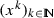is bounded, then

\begin{aligned} \lim _{k\rightarrow \infty }\mathop {\mathrm{dist}}\nolimits _{\omega (x^0)}(x^k)=0 \end{aligned}
(6.6)

and $$\omega (x^0)$$ is a nonempty, compact and connected subset of $$\mathop {\mathrm{zer}}\nolimits \partial \varphi$$ over which $$\varphi$$ is constant.

### Proof

(6.5) follows from (6.2), Proposition 3.4(ii) and 3.4(iv), and the fact that the sum of square-summable sequences is square summable.

Ifis bounded, that $$\omega (x^0)$$ is nonempty, compact and connected and $$\lim _{k\rightarrow \infty }\mathop {\mathrm{dist}}\nolimits _{\omega (x^0)}(x^k)=0$$ follow by [10, Lem.s 5(ii),(iii), Rem. 5]. That $$\varphi$$ is constant on $$\omega (x^0)$$ follows by a similar argument as in [10, Lem. 5(iv)]. $$\square$$

The following is [10, Lem. 6], therefore we state it with no proof.

### Lemma 6.9

(Uniformized KL property) Let $$K\subset \mathbb {R}^n$$ be a compact set and suppose that the proper lower semi-continuous function $$\varphi :\mathbb {R}^n\rightarrow \overline{{\mathbb {R}}}$$ is constant on K and satisfies the KL property at every $${x}^\star \in K$$. Then there exist $$\varepsilon >0$$, $$\eta >0$$, and a continuous concave function $$\psi :[0,\eta ]\rightarrow [0,+\infty )$$ such that properties 3.9(i), 3.9(ii) and 3.9(iii) hold, and

1. (iv’)

for all $$x_\star \in K$$ and x such that $$\mathop {\mathrm{dist}}\nolimits _K(x)<\varepsilon$$ and $$\varphi (x_\star )< \varphi (x)<\varphi (x_\star )+\eta$$,

\begin{aligned} \psi '(\varphi (x)-\varphi (x_\star ))\mathop {\mathrm{dist}}\nolimits (0,\partial \varphi (x))\ge 1. \end{aligned}
(6.7)

### Proof of Lemma 3.1

Let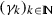be the sequence of stepsize parameters computed by

. To arrive to a contradiction, suppose that $$k_0$$ is the smallest element of $$\mathbb {N}$$ such that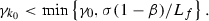Clearly, $$k_0 \ge 1$$. Moreover $$\sigma ^{-1}\gamma _{k_0}$$ must satisfy the condition in step 4: for some $$w\in \mathbb {R}^n$$ (corresponding to $$w^k=x^k+\tau _k d^k$$ selected before going back to step 1 after the condition in step 4 is passed, which might differ from the final value of $$w^k$$ after step 4 is passed)

\begin{aligned} \varphi (T_{\sigma ^{-1}\gamma _{k_0}}(w)) {}>{} \varphi _{\sigma ^{-1}\gamma _{k_0}}(w) {}-{} \frac{\beta \sigma ^{-1}\gamma _{k_0}}{2}\Vert R_{\sigma ^{-1}\gamma _{k_0}}(w)\Vert ^2. \end{aligned}

But from Proposition 2.2(ii) we also have

\begin{aligned} \varphi (T_{\sigma ^{-1}\gamma _{k_0}}(w)) {}\le {}&\varphi _{\sigma ^{-1}\gamma _{k_0}}(w) {}-{} \frac{\sigma ^{-1}\gamma _{k_0}}{2}(1-\sigma ^{-1}\gamma _{k_0}L_f)\Vert R_{\sigma ^{-1}\gamma _{k_0}}(w)\Vert ^2\\ {}\le {}&\varphi _{\sigma ^{-1}\gamma _{k_0}}(w) {}-{} \frac{\beta \sigma ^{-1}\gamma _{k_0}}{2}\Vert R_{\sigma ^{-1}\gamma _{k_0}}(w)\Vert ^2, \end{aligned}

where last inequality follows from $$\sigma ^{-1}\gamma _{k_0} < (1-\beta )/L_f$$. This leads to a contradiction, therefore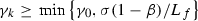as claimed. That $$\gamma _k$$ is asymptotically constant follows since the sequenceis nonincreasing. $$\square$$

### Proof of Proposition 3.4

We have

\begin{aligned} \varphi (x^{k+1})&\le \varphi _{\gamma _k}(w^k) - \tfrac{\beta \gamma _k}{2}\Vert R_{\gamma _k}(w^k)\Vert ^2 \nonumber \\&\le \varphi _{\gamma _k}(x^k) - \tfrac{\beta \gamma _k}{2}\Vert R_{\gamma _k}(w^k)\Vert ^2 \nonumber \\&\le \varphi (x^k)-\tfrac{\beta \gamma _k}{2}\Vert R_{\gamma _k}(w^k)\Vert ^2-\tfrac{{\gamma _k}}{2}\Vert R_{\gamma _k}(x^k)\Vert ^2, \end{aligned}
(6.8)

where the first inequality comes from step 4, the second from step 3 and the third from Proposition 2.2(i). This shows 3.4(i). Let $$\varphi _\star =\lim _{k\rightarrow \infty }\varphi (x^k)$$, which exists since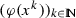is monotone. If $$\varphi _\star =-\infty$$, clearly $$\inf \varphi =-\infty$$ and $$\omega (x^0)=\emptyset$$ due to properness and lower semicontinuity of $$\varphi$$ and to the monotonic behavior of. Otherwise, telescoping the inequality we get

\begin{aligned} \frac{1}{2} \sum _{i=0}^k{ \gamma _i \left( \beta \Vert R_{\gamma _i}(w^i)\Vert ^2 {}+{} \Vert R_{\gamma _i}(x^i)\Vert ^2 \right) } {}\le {} \varphi (x^0) {}-{} \varphi (x^{k+1}) {}\le {} \varphi (x^0) {}-{} \varphi _\star \end{aligned}
(6.9)

and since $$\gamma _k$$ is uniformly lower bounded by a positive number (see Lemma 3.1) 3.4(ii) follows, hence 3.4(iii). If $$\beta >0$$, observing that for k large enough such that $$\gamma _k\equiv \gamma _\infty$$ we have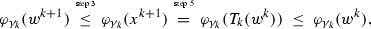similar argumentations as those for proving 3.4(ii) show 3.4(iv). $$\square$$

### Proof of Theorem 3.5

If $$\inf \varphi =-\infty$$ there is nothing to prove. Otherwise, since the sequenceis nonincreasing, from (6.9) we get

\begin{aligned} \frac{(k+1)\gamma _{k}}{2}\left( \min _{i=0\ldots k}\Vert R_{\gamma _{i}}(x^i)\Vert ^2 + \beta \min _{i=0\ldots k}\Vert R_{\gamma _{i}}(w^i)\Vert ^2\right) \le \varphi (x^0) - \inf \varphi . \end{aligned}

Rearranging the terms and invoking Lemma 3.1 gives the result. $$\square$$

### Proof of Theorem 3.6

The proof is similar to that of [15, Thm. 4]. By Proposition 2.5(iii) we know that $$\varphi _{\gamma }\le \varphi ^\gamma$$ for any $$\gamma >0$$. Combining this with (6.8) we get(6.10)

and in particular, for $$x_\star \in \mathop {\hbox {arg min}}\limits \varphi$$,

where the last inequality follows by convexity of $$\varphi$$. If $$\varphi (x^0)-\inf \varphi \ge R^2/{\gamma _0}$$, then the optimal solution of the latter problem for $$k=0$$ is $$\alpha =1$$ and we obtain (3.1). Otherwise, the optimal solution is

\begin{aligned} \alpha =\frac{{\gamma _{k}}(\varphi (x^k)-\inf \varphi )}{R^2}\le \frac{{\gamma _{k}}(\varphi (x^0)-\inf \varphi )}{R^2}\le 1, \end{aligned}

and we obtain

\begin{aligned} \varphi (x^{k+1})\le \varphi (x^k)-\frac{{\gamma _{k}}(\varphi (x^k)-\inf \varphi )^2}{2R^2}. \end{aligned}

Letting $$\lambda _k=\frac{1}{\varphi (x^k)-\inf \varphi }$$ the latter inequality is expressed as

\begin{aligned} \frac{1}{\lambda _{k+1}}\le \frac{1}{\lambda _k}-\frac{{\gamma _{k}}}{2R^2\lambda _{k+1}^2}. \end{aligned}

Multiplying both sides by $$\lambda _{k}\lambda _{k+1}$$ and rearranging

\begin{aligned} \lambda _{k+1}\ge \lambda _k+\frac{{\gamma _{k}}}{2R^2}\frac{\lambda _{k+1}}{\lambda _k}\ge \lambda _k+\frac{{\gamma _{k}}}{2R^2}, \end{aligned}

where the latter inequality follows from the fact thatis nonincreasing, cf. Proposition 3.4(i). Telescoping the inequality and using Lemma 3.1, we obtain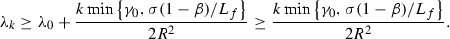Rearranging, we arrive at (3.2). $$\square$$

### Proof of Theorem 3.7

If (3.3) holds, then $$\varphi$$ has bounded level sets and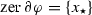. In particular, $$\omega (x^0)\ne \emptyset$$ and Proposition 3.4(iii) then ensures $$x^k\rightarrow x_\star$$. Therefore, there is $$k_0\in \mathbb {N}$$ such that $$x^k \in N$$ for all $$k\ge k_0$$. Inequality (6.10) holds, and in particular for $$k\ge k_0$$

where the second inequality follows by convexity of $$\varphi$$ and (3.3). The minimum of last expression is achieved for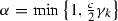. When $$\gamma _k < 2c^{-1}$$ we have the bound

\begin{aligned} \varphi (x^{k+1}) - \inf \varphi \le (1-\tfrac{c}{4}\gamma _k)(\varphi (x^k) - \inf \varphi ). \end{aligned}

When instead $$\gamma _k\ge 2c^{-1}$$ we have the bound

\begin{aligned} \varphi (x^{k+1}) - \inf \varphi \le (c\gamma _k)^{-1}(\varphi (x^k) - \inf \varphi ) \le \tfrac{1}{2}(\varphi (x^k) - \inf \varphi ). \end{aligned}

Therefore $$\varphi (x^{k+1}) - \inf \varphi \le \omega (\varphi (x^k) - \inf \varphi )$$, where

\begin{aligned} \omega&\le \sup _k \max \bigl \{\tfrac{1}{2}, 1-\tfrac{c}{4}\gamma _k \bigr \}\\&\le \max \bigl \{\tfrac{1}{2}, 1-\tfrac{c}{4}\min \{\gamma _0,\sigma (1-\beta )/L_f\} \bigr \} \in \bigl [\tfrac{1}{2},1\bigr ), \end{aligned}

last inequality following from Lemma 3.1. This proves the claim on the sequence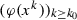and using inequality (6.8) the same holds for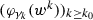. From the error bound (3.3) we obtain that $$x^k\rightarrow x_\star$$ R-linearly. If the same error bound holds for $$\varphi _{\gamma _\infty }$$, then also $$w^k\rightarrow x_\star$$ R-linearly. $$\square$$

### Proof of Theorem 3.10

The case where the sequence is finite does not deserve any further investigation, therefore we assume thatis infinite. We then assume that $$R_{\gamma _k}(x^k)\ne 0$$ which implies through Proposition 3.4 that $$\varphi (x^{k+1})<\varphi (x^k)$$. Due to (6.6), the KL property for $$\varphi$$, and Lemma 6.9, there exist $$\varepsilon ,\eta >0$$ and a continuous concave function $$\psi :[0,\eta ]\rightarrow [0,+\infty )$$ such that for all x with $$\mathop {\mathrm{dist}}\nolimits _{\omega (x^0)}(x)<\varepsilon$$ and $$\varphi ({x}^\star )< \varphi (x)<\varphi (x_\star )+\eta$$ one has

\begin{aligned} \psi '(\varphi (x)-\varphi (x_\star ))\mathop {\mathrm{dist}}\nolimits (0,\partial \varphi (x))\ge 1. \end{aligned}

According to Proposition 6.8 there exists a $$k_1\in \mathbb {N}$$ such that $$\mathop {\mathrm{dist}}\nolimits _{\omega (x^0)}(x^k)<\varepsilon$$ for all $$k\ge k_1$$. Furthermore, since $$\varphi (x^k)$$ converges to $$\varphi (x_\star )$$ there exists a $$k_2$$ such that $$\varphi (x^k)<\varphi (x_\star )+\eta$$ for all $$k\ge k_2$$. Take. Then for every $$k\ge \bar{k}$$ we have

\begin{aligned} \psi '(\varphi (x^k)-\varphi (x_\star ))\mathop {\mathrm{dist}}\nolimits (0,\partial \varphi (x^k))\ge 1. \end{aligned}

From Proposition 3.4(i)

\begin{aligned} \varphi (x^{k+1})\le \varphi (x^k)-\tfrac{\beta \gamma _k}{2}\Vert R_{\gamma _k}(w^k)\Vert ^2. \end{aligned}

For every $$k>0$$ let $$\tilde{\nabla }\varphi (x^k) {}={} \nabla f(x^{k})-\nabla f(w^{k-1})+R_{\gamma _{k-1}}(w^{k-1})$$. Since $$R_{\gamma _{k-1}}(w^{k-1}) {}\in {} \nabla f(w^{k-1}) + \partial g(x^k)$$, then $$\tilde{\nabla }\varphi (x^k) {}\in {} \partial \varphi (x^k)$$ and

\begin{aligned} \Vert \tilde{\nabla }\varphi (x^k)\Vert {}\le {}&\Vert \nabla f(x^{k})-\nabla f(w^{k-1})\Vert {}+{} \Vert R_{\gamma _{k-1}}(w^{k-1})\Vert \\ {}={}&(1+{\gamma _{k-1}} L_f) \Vert R_{\gamma _{k-1}}(w^{k-1})\Vert . \end{aligned}

From (6.7)

\begin{aligned} \psi '(\varphi (x^{k})-\varphi (x_\star )) {}\ge {} \frac{1}{\Vert \tilde{\nabla }\varphi (x^k)\Vert } {}\ge {} \frac{1}{(1+{\gamma _{k-1}} L_f) \Vert R_{\gamma _{k-1}}(w^{k-1})\Vert }. \end{aligned}

Let $$\Delta _k= \psi (\varphi (x^{k})-\varphi (x_\star ))$$. By concavity of $$\psi$$ and Proposition 3.4(i)

\begin{aligned} \Delta _k-\Delta _{k+1}&\ge \psi '(\varphi (x^{k})-\varphi (x_\star ))(\varphi (x^k)-\varphi (x^{k+1}))\\&\ge \frac{\beta \gamma _k}{2 (1+\gamma _{k-1} L_f)}\frac{\Vert R_{\gamma _{k}}(w^k)\Vert ^2}{\Vert R_{\gamma _{k-1}}(w^{k-1})\Vert }\\&\ge \frac{\beta \gamma _{\min }}{2 (1+\gamma _0 L_f)}\frac{\Vert R_{\gamma _{k}}(w^k)\Vert ^2}{\Vert R_{\gamma _{k-1}}(w^{k-1})\Vert } \end{aligned}

where, see Lemma 3.1, or

\begin{aligned} \Vert R_{\gamma _{k}}(w^{k})\Vert ^2\le \alpha (\Delta _k-\Delta _{k+1})\Vert R_{\gamma _{k-1}}(w^{k-1})\Vert \end{aligned}
(6.11)

where $$\alpha = 2 (1+\gamma _0 L_f)/(\beta \gamma _{\min })$$. Applying Lemma 6.7 with

\begin{aligned} \delta _k=\alpha \Delta _k,\quad \beta _k=\Vert R_{\gamma _{k-1}}(w^{k-1})\Vert , \end{aligned}

we conclude that $$\sum _{k=0}^\infty \Vert R_{\gamma _{k}}(w^{k})\Vert <\infty$$. From (6.3), using the fact that $$\gamma _k\le \gamma _0$$ for all k, then it follows that

\begin{aligned} \sum _{k=0}^{\infty }\Vert x^{k+1}-x^k\Vert <\infty . \end{aligned}

Thenis a Cauchy sequence, hence it converges to a point that, by Proposition 3.4, is a critical point $$x_\star$$ of $$\varphi$$. $$\square$$

### Proof of Theorem 3.11

Theorem 3.10 ensures thatconverges to a critical point, be it $$x_\star$$. We know from Lemma 3.1 that eventually $$\gamma _k = \gamma _\infty > 0$$, therefore we assume k is large enough for this purpose and indicate $$\gamma$$ in place of $$\gamma _k$$ for simplicity. Denoting $$A_k=\sum _{i=k}^\infty \Vert x^{i+1}-x^i\Vert$$ clearly $$A_k \ge \Vert x^k-x_\star \Vert$$, so we will prove that $$A_k$$ converges linearly to zero to obtain the result. Note that by (6.3) we know that

\begin{aligned} \Vert x^{i+1}-x^i\Vert \le \gamma \Vert R_{\gamma }(w^i)\Vert + \bar{\tau } c (1+\gamma L_f)\Vert R_{\gamma }(w^{i-1})\Vert . \end{aligned}

Therefore we can upper bound $$A_k$$ as follows

\begin{aligned} A_k {}\le {}&\textstyle \bar{\tau } c (1+\gamma L_f)\Vert R_{\gamma }(w^{k-1})\Vert {}+{} \left( \gamma + \bar{\tau }c(1+\gamma L_f) \right) \sum _{i=k}^\infty { \Vert R_{\gamma }(w^i)\Vert } \nonumber \\ {}\le {}&\textstyle \left( \gamma + \bar{\tau }c(1+\gamma L_f) \right) \sum _{i=k-1}^\infty { \Vert R_{\gamma }(w^i)\Vert , } \end{aligned}
(6.12)

and reduce the problem to proving linear convergence of $$B_k = \sum _{i=k}^\infty \Vert R_{\gamma }(w^i)\Vert$$. When $$\psi$$ is as in (3.4), for sufficiently large k the KL inequality reads

\begin{aligned} \varphi (x^k)-\varphi (x_\star ) \le [\sigma (1-\theta )\Vert v^k\Vert ]^{\frac{1}{\theta }},\quad \forall v^k\in \partial \varphi (x^k). \end{aligned}

Taking $$v^k = \nabla f(x^k) - \nabla f(w^{k-1}) + R_{\gamma }(w^{k-1}) \in \partial \varphi (x^k)$$, this in turn yields

\begin{aligned} \varphi (x^k)-\varphi (x_\star ) \le \left[ \sigma (1-\theta )(1+{\gamma } L_f)\Vert R_{\gamma }(w^{k-1})\Vert \right] ^{\frac{1}{\theta }}, \end{aligned}
(6.13)

(see the proof of Theorem 3.10). Inequality (6.11) holds, for sufficiently large k, with $$\Delta _k = \sigma (\varphi (x^k)-\varphi (x_\star ))^{1-\theta }$$ in this case. Applying Lemma 6.7 with

\begin{aligned} \delta _k=\alpha \Delta _{k},\quad \beta _k=\Vert R_{\gamma }(w^{k-1})\Vert =B_{k-1}-B_k, \end{aligned}

we obtain

\begin{aligned} B_k&\le (B_{k-1} - B_k) + \sigma (\varphi (x^k)-\varphi (x_\star ))^{1-\theta } \\&\le (B_{k-1} - B_k) + \sigma \left[ \sigma (1-\theta )(1+{\gamma } L_f)(B_{k-1} - B_k)\right] ^{\frac{1-\theta }{\theta }}, \end{aligned}

where the second inequality is due to (6.13). Since $$B_{k-1}-B_k \rightarrow 0$$, then for k large enough it holds that $$\sigma (1+{\gamma } L_f)(B_{k-1}-B_k) \le 1$$, and the last term in the previous chain of inequalities is increasing in $$\theta$$ when $$\theta \in (0,\tfrac{1}{2}]$$. Therefore $$B_k$$ eventually satisfies

\begin{aligned} B_k \le C(B_{k-1}-B_k), \end{aligned}

where $$C>0$$, and so $$B_k \le [C/(1+C)] B_{k-1}$$, i.e., $$B_k$$ converges to zero Q-linearly. This in turn implies that $$\Vert x^k-x_\star \Vert$$ converges to zero with R-linear rate. Furthermore,

\begin{aligned} \Vert w^k-x_\star \Vert&= \Vert x^k - x_\star + \tau _k d^k\Vert \\&\le \Vert x^k-x_\star \Vert + \bar{\tau }c\Vert R_{\gamma _k}(x^k)\Vert \\&= \Vert x^k-x_\star \Vert + \bar{\tau }c{\gamma _k}^{-1}\Vert T_{\gamma _k}(x^k)-x^k\Vert \\&\le (1+\bar{\tau }c\gamma _k^{-1})\Vert x^k-x_\star \Vert + \bar{\tau }c{\gamma _k}^{-1}\Vert T_{\gamma _k}(x^k)-T_{\gamma _k}(x_\star )\Vert \\&\le (1+\bar{\tau }c\gamma _k^{-1})\Vert x^k-x_\star \Vert + \bar{\tau }c{\gamma _k}^{-1}\Vert x^k - \gamma _k\nabla f(x^k)- x_\star + \gamma _k\nabla f(x_\star )\Vert \\&\le (1+\bar{\tau }c(2\gamma _k^{-1} + L_f))\Vert x^k-x_\star \Vert , \end{aligned}

where the last two inequalities follow by nonexpansiveness of $$\mathop {\mathrm{prox}}\nolimits _{\gamma g}$$ and Lipschitz continuity of $$\nabla f$$. Since $$\gamma _k$$ is lower bounded by a positive quantity, then we deduce that also $$\Vert w^k-x_\star \Vert$$ converges R-linearly to zero. $$\square$$

### Proof of Theorem 4.1

Since $$w^k = x^k - B_k^{-1}\nabla \varphi _{\gamma }(x^k)$$, letting $$k\rightarrow \infty$$ and using (4.1) we have that

\begin{aligned} 0 {}\leftarrow {} \frac{(B_k - \nabla ^2\varphi _{\gamma }(x_\star ))(w^k-x^k)}{\Vert w^k-x^k\Vert } {}={}&{}-\frac{\nabla \varphi _{\gamma }(x^k) + \nabla ^2\varphi _{\gamma }(x_\star )(w^k-x^k)}{\Vert w^k-x^k\Vert }\\ {}={}&{}-\frac{\nabla \varphi _{\gamma }(x^k) - \nabla \varphi _{\gamma }(w^k) + \nabla ^2\varphi _{\gamma }(x_\star )(w^k-x^k)}{\Vert w^k-x^k\Vert }\\&{}-\frac{\nabla \varphi _{\gamma }(w^k)}{\Vert w^k-x^k\Vert }. \end{aligned}

By strict differentiability of $$\nabla \varphi _{\gamma }$$ at $$x_\star$$ we obtain

\begin{aligned} \lim _{k\rightarrow \infty }{ \frac{ \Vert \nabla \varphi _{\gamma }(w^k)\Vert }{ \Vert w^k-x^k\Vert } } {}={} 0. \end{aligned}
(6.14)

By nonsingularity of $$\nabla ^2\varphi _{\gamma }(x_\star )$$ and since $$w^k\rightarrow x^\star$$, there exists $$\alpha >0$$ such that $$\Vert \nabla \varphi _{\gamma }(x^k)\Vert \ge \alpha \Vert x^k-x_\star \Vert$$ for k large enough. Therefore, for k sufficiently large,

\begin{aligned} \frac{ \Vert \nabla \varphi _{\gamma }(w^k)\Vert }{ \Vert w^k-x^k\Vert } {}\ge {} \frac{ \alpha \Vert w^k-x_\star \Vert }{ \Vert w^k-x^k\Vert } {}\ge {} \frac{ \alpha \Vert w^k-x_\star \Vert }{ \Vert w^k-x_\star \Vert +\Vert x^k-x_\star \Vert }. \end{aligned}

Using (6.14) we get

\begin{aligned} \lim _{k\rightarrow \infty }{ \frac{ \Vert w^k-x_\star \Vert }{ \Vert w^k-x_\star \Vert +\Vert x^k-x_\star \Vert } } {}={} \lim _{k\rightarrow \infty }{ \frac{ \Vert w^k-x_\star \Vert /\Vert x^k-x_\star \Vert }{ \Vert w^k-x_\star \Vert /\Vert x^k-x_\star \Vert +1 } } {}={} 0, \end{aligned}

from which we obtain

\begin{aligned} \lim _{k\rightarrow \infty }{ \frac{\Vert w^k-x_\star \Vert }{\Vert x^k-x_\star \Vert } } {}={} 0. \end{aligned}
(6.15)

Finally,

\begin{aligned} \Vert x^{k+1}-x_\star \Vert {}={}&\Vert T_{\gamma }(w^k)-T_{\gamma }(x_\star )\Vert \nonumber \\ {}={}&\left\| \mathop {\mathrm{prox}}\nolimits _{\gamma g}(w^k-\gamma \nabla f(w^k)) {}-{} \mathop {\mathrm{prox}}\nolimits _{\gamma g}(x_\star -\gamma \nabla f(x_\star )) \right\| \nonumber \\ {}\le {}&\left\| w^k - \gamma \nabla f(w^k) {}-{} x_\star + \gamma \nabla f(x_\star ) \right\| \nonumber \\ {}\le {}&(1+\gamma L_f)\Vert w^k-x_\star \Vert , \end{aligned}
(6.16)

where the first inequality follows from nonexpansiveness of $$\mathop {\mathrm{prox}}\nolimits _{\gamma g}$$ and the second from Lipschitz continuity of $$\nabla f$$. Using (6.16) in (6.15) we obtain thatandconverge Q-superlinearly to $$x_\star$$. $$\square$$

### Proof of Theorem 4.2

From Proposition 6.4(a) it follows that $$\nabla \varphi _{\gamma }$$ is strictly differentiable and continuously semidifferentiable at $$x_\star$$. Moreover, we know from Lemma 3.1 that eventually $$\gamma _k = \gamma _\infty > 0$$. Therefore we assume that k is large enough for this purpose and indicate $$\gamma$$ in place of $$\gamma _k$$ for simplicity. We denote for short $$g^k = \nabla \varphi _{\gamma }(x^k)$$. In

\begin{aligned} w^k-x^k = \tau _k d^k = -\tau _k B_k^{-1}g^k, \end{aligned}

and by (4.1) and Cauchy-Schwarz inequality

\begin{aligned} \frac{\Vert (B_k-\nabla ^2\varphi _{\gamma }(x_\star ))(w^k-x^k)\Vert }{\Vert w^k-x^k\Vert }&= \frac{\Vert g^k+\nabla ^2\varphi _{\gamma }(x_\star )d^k\Vert }{\Vert d^k\Vert } \\&\ge \left| \frac{\langle d^k,g^k+\nabla ^2\varphi _{\gamma }(x_\star )d^k \rangle }{\Vert d^k\Vert ^2}\right| \rightarrow 0. \end{aligned}

Therefore

\begin{aligned} -\langle g^k,d^k \rangle = \langle d^k,\nabla ^2\varphi _{\gamma }(x_\star )d^k \rangle + o(\Vert d^k\Vert ^2). \end{aligned}
(6.17)

Since $$\nabla ^2\varphi _{\gamma }(x_\star )$$ is positive definite, there is $$\eta >0$$ such that for sufficiently large k

\begin{aligned} - \langle g^k,d^k \rangle \ge \eta \Vert d^k\Vert ^2. \end{aligned}
(6.18)

Sinceis continuous at $$x_\star$$ and $$x^k\rightarrow x_\star$$, we have(6.19)

Next, since $$x^k\rightarrow x_\star$$, for k large enough $$\nabla \varphi _{\gamma }$$ is semidifferentiable at $$x^k$$ and we can expand $$\varphi _{\gamma }$$ around $$x^k$$ using [31, Ex. 13.7(c)] to obtain

where the second equality is due to (6.19), and the last equality is due to (6.17). Therefore, using (6.18), for sufficiently large k

\begin{aligned} \varphi _{\gamma }(x^k+d^k) - \varphi _{\gamma }(x^k) \le -\tfrac{\eta }{2}\Vert d^k\Vert ^2 < 0, \end{aligned}

i.e., $$\tau _k = 1$$ satisfies the non-increase condition. As a consequence,

eventually reduces to the iterations of Theorem 4.1 and the proof follows. $$\square$$

### Proof of Theorem 4.3

Suppose that Assumption 6(i) holds. Since $$x_\star \in \mathop {\mathrm{zer}}\nolimits \partial \varphi$$ and $$\nabla ^2\varphi _{\gamma }(x_\star ) \succ 0$$, it follows that $$x_\star$$ is a strong local minimizer of $$\varphi _{\gamma }$$, hence of $$\varphi$$ in light of Proposition 2.2(i) and 2.3(i). Theorem 3.7 then ensures thatandconverge linearly to $$x_\star$$. If instead Assumption 6(ii) holds, then we can invoke Theorem 3.11 (since $$\Vert \nabla \varphi _{\gamma _k}(x^k)\Vert \le (1+\gamma _0L_f)\Vert R_{\gamma _k}(x^k)\Vert$$) to infer thatandconverge linearly to a critical point, be it $$x_\star$$. In both cases we can apply Proposition 6.5 and for k sufficiently large(6.20)

Since the convergence is linear, then the right-hand side of (6.20) is summable. With similar arguments to those of [26, Lem. 3.2] we can see that eventually $$\langle s^k,y^k \rangle >0$$. Therefore we can apply [70, Thm. 3.2], which ensures that condition (4.1) holds. The result follows then from Theorem 4.2. $$\square$$

## Rights and permissions

Reprints and Permissions